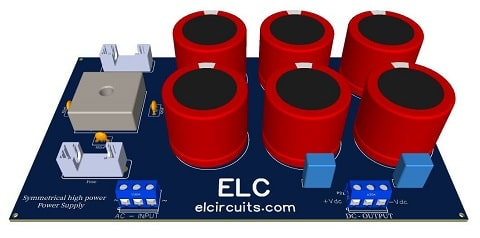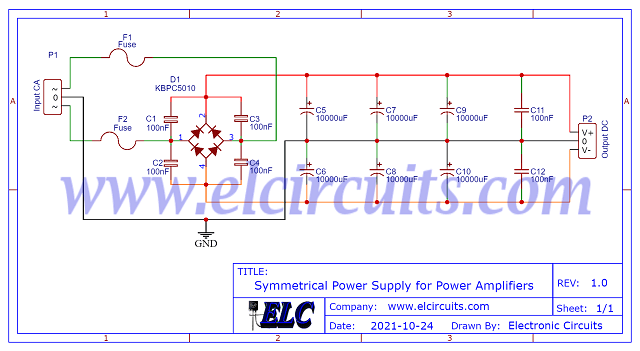## Monday, November 29, 2021Fig. 1 - Symmetrical Power Supply for Power Amplifiers

For power amplifier lovers, who build their own audio power amplifiers, here is a good full wave rectifier linear symmetric power supply that will meet the power demand without leaving anything to be desired in terms of stability.

This power supply is designed for amplifiers with power up to 2500W, it will work without any problems with great stability.

Most power amplifier circuits require a symmetrical power supply, and what differs from each other is always the power required from the supply.

As we know, a good power supply with good filtering will determine the quality and final power of your amplifier.

### You may be interested in:

The complete schematic diagram of the power supply is shown below in Figure 2, it is a simple but complete power supply.Fig.2 - Electrical schematic Symmetrical Power Supply for Power Amplifiers

However, it is worth remembering that for each amplifier power range, we can assemble a type of power supply according to the power of your amplifier.

We are presenting 3 different configurations to exemplify the different types of power amplifiers.

There are 3 examples of simplified formulas for you to calculate the  power supply Current and Voltage according to the power of your amplifier.

Remembering that the PCB printed circuit board is the same for all configurations.

## Configuration 1:

In this configuration, we can use amplifiers that have a total power up to 400W

We need to calculate the maximum power supply current, considering 45V supply, and the maximum power of 400W. Calculating ohms Law: P = V * I
• I = P / V
• I = 400/45
• I = 8.88A
Now you need to stipulate the maximum ripple allowed in your design, in this case: If you set the maximum ripple voltage to 5%!
• V_ripple = V_ps * 5%
• V_ripple = 45V * 5%
• V_ripple  = 2.25V
Once the maximum ripple voltage has been stipulated, we need to calculate the capacitor for that ripple at 5% of the source, "You may be calculating the percentage that best suits your design."

Capacitor Calculation Formula : C = I / F * V_ripple
• C = 8.88 / 120 * 2.25
• C = 8.88 / 270
• C = 0.032888 = > C = 32.888X10^-6 = 32.888uF
As our board was designed to support 6 capacitors. We can divide the entire value into uF and divide by 6 Capacitors.
• C_individual = 32.888 / 6
• C_individual  = 5.481uF

For a closer commercial capacitors value, we have:
C_individual = 6.800uF / 63V

• 6 x 6.800uF Capacitor
• 15A rectifier bridge

## Configuration 2:

In this configuration, we can use amplifiers that have a total power up to 1200W

We need to calculate the maximum power supply current, considering 75V supply, and the  maximum power of 1200W. Calculating ohms Law: P = V * I
• I = P / V
• I = 1200/75
• I = 16A

Now you need to stipulate the maximum ripple allowed in your design, in this case: If you set the maximum ripple voltage to 5%!
• V_ripple = V_ps * 5%
• V_ripple = 75V * 5%
• V_ripple  = 3.75V

Once the maximum ripple voltage has been stipulated, we need to calculate the capacitor for that ripple at 5% of the source, "You may be calculating the percentage that best suits your design."

Capacitor Calculation Formula : V_ripple = I / F * C
• C = I / F * V_ripple
• C = 16 / 120 * 3.75
• C = 16 / 450
• C = 0.035555 = > C = 35.555X10^-6 = 35.555uF

As our board was designed to support 6 capacitors. We can divide the entire value into uF and divide by 6 Capacitors.
• C_individual = 35.555 / 6
• C_individual  = 5.925uF

For a closer commercial capacitors value, we have:
C_individual = 6.800uF / 100V

• 6 x 6.800uF Capacitor
• 25A rectifier bridge

## Configuration 3:

In this configuration, we can use amplifiers that have a total power up to 2500W

We need to calculate the maximum power supply current, considering 95V supply, and the maximum power of 2500W. Calculating ohms Law: P = V * I
• I = P / V
• I = 2500/95
• I = 26A

Now you need to stipulate the maximum ripple allowed in your design, in this case: If you set the maximum ripple voltage to 5%!
• V_ripple = V_ps * 5%
• V_ripple = 95V * 5%
• V_ripple  = 4.75V

Once the maximum ripple voltage has been stipulated, we need to calculate the capacitor for that ripple at 5% of the source, "You may be calculating the percentage that best suits your design."

Capacitor Calculation Formula : C = I / F * V_ripple
• C = 26 / 120 * 4.75
• C = 26 / 570
• C = 0.045614 = > C = 45.614X10^-6 = 45.614uF
As our board was designed to support 6 capacitors. We can divide the entire value into uF and divide by 6 Capacitors.
• C_individual = 45.614 / 6
• C_individual  = 7.602uF

For a closer commercial capacitors value, we have:
C_individual = 10.000uF / 200V

• 6 x 10.000uF Capacitor
• 40A rectifier bridge